Body volume - examples - page 16

1. Fuel economyHow many kilometers is sufficient petrol in the cylinder fuel tank with a diameter 40 cm and the base of tank length 1 m, when it is filled to 60% and if the car consume 15 liters per 100 km?
2. Wood lumberWooden lumber is 4 m long and has a cross section square with side 15 cm. Calculate: a) the volume of lumber b) the weight of the lumber if 1 m3 weighs 790 kg
3. TankTo cuboid tank whose bottom has dimensions of 9 m and 15 m were flow 1080 hectoliters of water. This was filled 40% of the tank volume. Calculate the depth of the tank.
4. Common cylinderI've quite common example of a rotary cylinder. Known: S1 = 1 m2, r = 0.1 m Calculate : v =? V =? You can verify the results?
5. PoolThe pool has the shape of a cylinder with a diameter 4.6m, the presumed height is 130cm. The bucket fits 12l of water. How many buckets do you have to bring to fill this pool?
6. Hexagonal prism 2The regular hexagonal prism has a surface of 140 cm2 and height of 5 cm. Calculate its volume.
7. Water level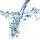To cuoid shaped poll, bottom size 2m and 3.5m; flows water at a rate of 50 liters per minute. How long will take to water reach level 50 cm?
8. CubeOne cube has edge increased 5 times. How many times will larger its surface area and volume?
9. Clay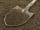How many cubic centimeters of clay is in a pit of dimensions 4 m x 3 m x 3 m?
10. Cuboid edges in ratioCuboid edges lengths are in ratio 2:4:6. Calculate their lengths if you know that the cuboid volume is 24576 cm3.
11. Brick wall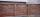Garden 70 m long and 48 m wide should surround with wall 2.1 meters high and 30 cm thick. Wall will be built on the garden ground. How many will we need bricks if to 1 m³ is required approximately 300 bricks?
12. Roof 8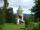How many liters of air are under the roof of tower which has the shape of a regular six-sided pyramid with a 3,6-meter-long bottom edge and a 2,5-meter height? Calculate the supporting columns occupy about 7% of the volume under the roof.
13. Cuboid enlargementBy how many percent increases the volume of cuboid if its every dimension increases by 30%?
14. Cuboid surfaceDetermine surface area of cuboid if its volume is 52.8 cm cubic and length of the two edges are 2 cm and 6 cm.
15. Water reservoir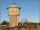The reservoir shape of a cylinder having a diameter 8 m and a height 2.8 m. The device indicates that the reservoir contains 800 hectoliters of water. Calculate how much % of volume is used.
16. Axial cutThe cone surface is 388.84 cm2, the axial cut is an equilateral triangle. Find the cone volume.A domed stadium is in the shape of spherical segment with a base radius of 150 m. The dome must contain a volume of 3500000 m³. Determine the height of the dome at its centre to the nearest tenth of a meter.Find the volume and surface of a regular quadrilateral pyramid if the bottom edge is 45 cm long and the pyramid height is 7 cm.How many liters of water fit into the shape of a cylinder with a bottom diameter 20 cm and a height 45 cm?Cuboid has a surface of 516 cm2. Side a = 6 cm and b = 12 cm. How long is the side c =?